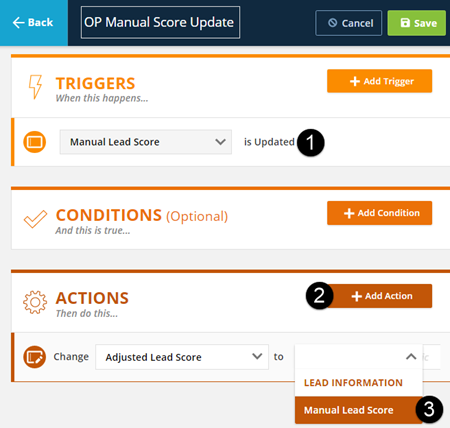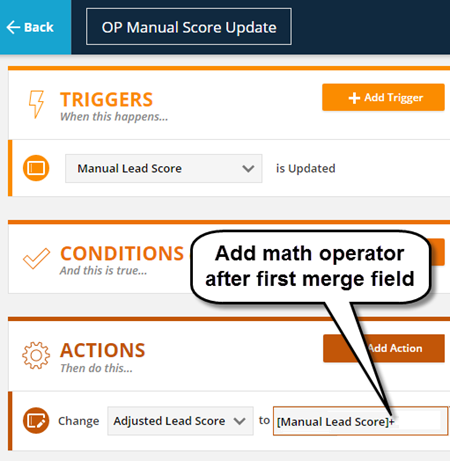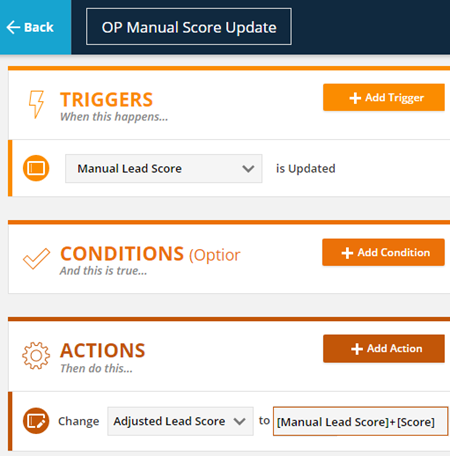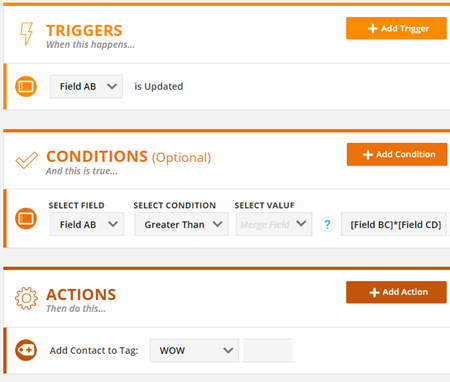searchUpdated

# Math Equations in Numeric and Price Fields

Applies to: All ONTRAPORT accounts.

You can create equations for numeric (integer) and price (currency) fields in Rules. The equations use merge fields provided in a drop down box to allow standard math operators to add, subtract, multiply or divide one numeric or price field by another. Whole numbers can also be used in the equations in numeric fields, and decimal numbers with up to two decimal places can be used in price fields.

Numeric fields round to the nearest whole number and do not allow for floating point or decimals. Price fields round to the nearest two decimal places.

Two usecases are provided below. One shows a custom lead scoring configuration for a customer who wants his team's personal interactions with a client added to ONTRAPORT's built in lead Score. The other usecase shows the use of an equation as a condition statement to multiply two numeric fields, compare the result against the value of a third numeric field, and Tag a Contact accordingly.

## Using Math Equations

In our first example, the business owner wants to be able to use a different lead score than the built in Score field. He added two custom fields, Manual Lead Score and Adjusted Lead Score, that are both numeric fields. His team will update the Manual Lead Score field whenever they speak to the customer on the phone and use the Adjusted Lead Score field as a guide to whom they need to call next.

After creating the fields, he creates a single global Rule. The trigger for the Rule is that the Manual Lead Score field is updated. He chooses the action Change the value of a field and specifies the Adjusted Lead Score field. First, he chooses Manual Lead Score from the drop down box:In this case, the account owner wants to add the Manual Lead Score to the system Score, so he adds a plus sign after the first merge field:All that is left to do is add the next merge field, Score, and save the Rule.Whenever the Manual Lead Score field is updated, the Rule updates the Adjusted Score Field with the sum of the Manual Score Field plus the Score field. The business owner's team can now prioritize their next calls by the Adjusted Lead Score field. The business owner can refine this system more by having a global Rule that looks for a change in the Adjusted Lead Score field and, if less than a certain number, assigns a Task to call the Contact.

### Usecase: Tag According to Multiple Values

A common usecase is to Tag a Contact based on the value of a field. A single numeric field can be tested against any combination of equal to or greater than / less than conditions. But multiple numeric fields can also be used with math operators.

In this case, our business owner wants to Tag a Contact where Field A is greater than Field B times Field C. He inserts the merge field for Field B, adds the multiplication symbol (the asterik), and then inserts the merge field for Field C. The Rule looks like this:## Valid Equations

In addition to standard addition, subtraction, multiplication and division operators, equations can be enclosed in parenthesis to force the order of operations.

• [Your Field]+1 - Increase the value of a numeric or price field by 1
• [Your Field]*4 - Multiply the value of a numeric or price field by 4
• 8/[Your Field] - Divide 8 by the value of a numeric or price field
• [Field A]*[Field B] - Multiply the value of a numeric field by the value of another numeric field, or multiply the value of a price field by the value of another price field. You should not mix numeric and price fields in the same equation.
• [Field A]*[Field B]/([Field C]+5) - This is a more complex equation using parenthesis to force the order of operations: Add 5 to the value of numeric field first, then use standard order of operations to multiply the value of a numeric field by the value of another numeric field and divide the result by the sum of the first operation. Avoid mixing numeric and price fields in the same equation to prevent flashbacks to 9th grade algebra homework.

Note: Numeric fields in ONTRAPORT round to the nearest whole number. Price fields round to the nearest decimal value to two decimal places. Errors, such as "divide by zero" errors are not reported and the values not updated.

### Articles in this section

Created - Updated
2 out of 2 found this helpful
Have more questions? Submit a request

•Jamie Mather

This is great and saves me time writing code at the application end to sum.count and divide field values before pushing them in to Ontrport. Do these formulas only work on number fields or currency fields also?

•Frank Hagan

Edit 8/20/2018 - We have added the same ability  to Price (currency) type fields. The article was updated to reflect this.

Hi Jamie - at this time the math operations are only available for numeric fields. We do have an active request to add "date math" in our Feature Request forums, but I didn't see a similar request for currency / price fields. Feel free to add the request there and our developers will take a look!

Edited by Frank Hagan
•Charles Dugan

we're putting together a custom quote object, and need to be able to calculate prices - qty x unit price - to the penny, not rounded to the nearest whole number:). when will formula's work in currency fields?

•Doug Freeman

The arithmetic functionality is great to have, but in some cases is mitigated by inability to then display the outcome as a 'readable' number in a message; ie, with commas & decimal places. Request is in to add this formatting change so if you're here and frustrated by same lack of functionality, please go in to Request List and vote.

•Frank Hagan

We have added the ability to use math equations on price (currency) fields as well. Price fields calculate numbers out to two decimal points as opposed to rounding to the nearest whole number. The article has been updated to show this.